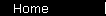### Gordon Growth Model

Valuation formula holding that the total return of a stock investment will equal its dividend yield plus its dividend growth rate:

R   =   D/P + G

where

D is next year's annual dividend;

P is the current share price;

G is the growth rate.

For the origin of the equation, see the "constant growth case" formula near the bottom of this stock valuation page - but think "dividends" where the page says "earnings". (Or if you're more of a people-person, you can read about the career of Myron J. Gordon.)

 home  |  glossary  |  calculator  |  about us  |  books